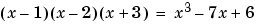MATLAB Function Referencecompan

Companion matrix

Syntax

• ````A` `=` ```compan(u)
``````

Description

```A = compan(u) ``` returns the corresponding companion matrix whose first row is `-u(2:n)/u(1)`, where `u` is a vector of polynomial coefficients. The eigenvalues of `compan(u)` are the roots of the polynomial.

Examples

The polynomialhas a companion matrix given by

• ```u = [1  0  -7  6]
A = compan(u)
A =
0    7   -6
1    0    0
0    1    0
```

The eigenvalues are the polynomial roots:

• ```eig(compan(u))

ans =
-3.0000
2.0000
1.0000
```

This is also `roots(u)`.

See Also

`eig`, `poly`, `polyval`, `roots`commandwindow compass© 1994-2005 The MathWorks, Inc.